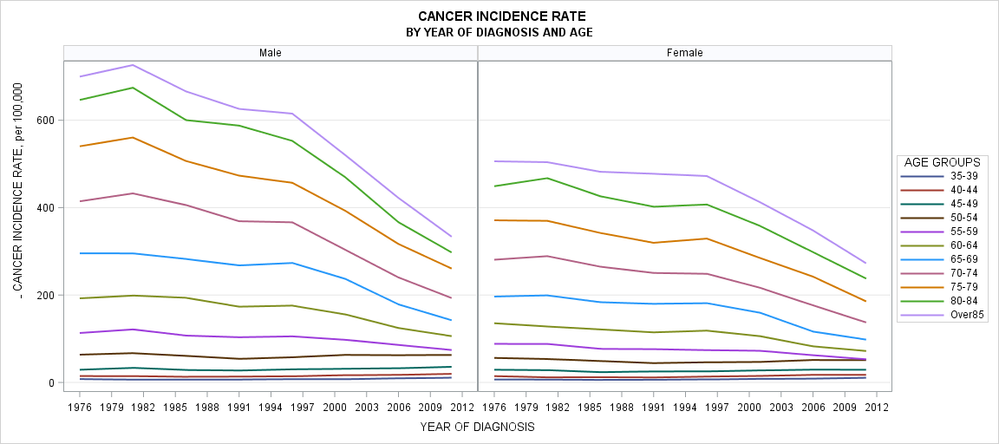## Plot x-axis is not formatted

Is there any way to force the plot to take up on formatting on X-axis display? I'd like to show x axis as formatted for P as a range of time periods. S=Gender and A-Age formats taken up no problem. Do you know why and how to fix it?``````proc format;
value P
1976="1976-1980"
1981="1981-1985"
1986="1986-1990"
1991="1991-1995"
1996="1996-2000"
2001="2001-2005"
2006="2006-2010"
2011="2011-2015"
;
value A
0 ="0-4"
5 ="5-9"
10="10-14"
15="15-19"
20="20-24"
25="25-29"
30="30-34"
35="35-39"
40="40-44"
45="45-49"
50="50-54"
55="55-59"
60="60-64"
65="65-69"
70="70-74"
75="75-79"
80="80-84"
85="Over85"
;
value S
1="Male"
2="Female";
;
run;

ods graphics / height=800 width=1800;
proc sgpanel data=mydata(where=(A>30));
series y=rate x=P/ group=A lineattrs=(pattern=solid) lineattrs=(thickness=2);
keylegend/title="AGE GROUPS" position=right;
format A A. S S. P P.;
colaxis label='YEAR OF DIAGNOSIS' fitpolicy=thin valuesformat=best4.0 values=(1980 to 2016 by 1);
rowaxis label='INCIDENCE RATE, per 100,000' grid;
title1 "INCIDENCE RATE";
title2 "BY YEAR OF DIAGNOSIS AND AGE";
run;``````

1 ACCEPTED SOLUTION

Accepted Solutions

## Re: Plot x-axis is not formatted

In addition to the plot type issues I think you have defined your P format incorrectly to begin with.

I think you intended

```proc format;
value P
1976 - 1980="1976-1980"
<etc>```

if you wanted a grouping format.

And since you seem to want to combine the values then perhaps you should do that in a separate summary procedure or data step to create the appropriate Y axis value(s) using the P format to group the years. Since you have a rate displayed you probably cannot get the graphing procedure to combine things across years correctly (averaging rates in general yield incorrect results unless the denominator is identical for each and every group of data).

3 REPLIES 3

## Re: Plot x-axis is not formatted

Interesting problem. There is an inconsistency in your variable names (you use X=P1 but set FORMAT P P.), but that doesn't seem to be the issue. For anyone interested in looking into this issue, here is some sample data:

``````data mydata;
do S = 1 to 2;
do A = 0 to 85 by 5;
do P = 1976 to 2011 by 5;
rate = A + P; output;
end;
end;
end;
run;

ods graphics / height=800 width=1800;
proc sgpanel data=mydata(where=(A>30));
series y=rate x=P/ group=A lineattrs=(pattern=solid) lineattrs=(thickness=2);
keylegend/title="AGE GROUPS" position=right;
format A A. S S. P P.;
colaxis label='YEAR OF DIAGNOSIS' fitpolicy=rotate;
rowaxis label='INCIDENCE RATE, per 100,000' grid;
title1 "INCIDENCE RATE";
title2 "BY YEAR OF DIAGNOSIS AND AGE";
run;``````DanH_sas
SAS Super FREQ

## Re: Plot x-axis is not formatted

I think what you want here is a VLINE chart, not a SERIES plot. The axis for the SERIES is continuous by default, which is probably why the format did not show up. Also, the nature of your format is going to require some kind of aggregation (probably mean). Try replacing the SERIES statement with this statement and see if you get what you want:

vline p / response=rate group=A stat=mean lineattrs=(pattern=solid) lineattrs=(thickness=2);

Hope this helps!

Dan

## Re: Plot x-axis is not formatted

In addition to the plot type issues I think you have defined your P format incorrectly to begin with.

I think you intended

```proc format;
value P
1976 - 1980="1976-1980"
<etc>```

if you wanted a grouping format.

And since you seem to want to combine the values then perhaps you should do that in a separate summary procedure or data step to create the appropriate Y axis value(s) using the P format to group the years. Since you have a rate displayed you probably cannot get the graphing procedure to combine things across years correctly (averaging rates in general yield incorrect results unless the denominator is identical for each and every group of data).

Discussion stats
• 3 replies
• 564 views
• 4 likes
• 4 in conversation